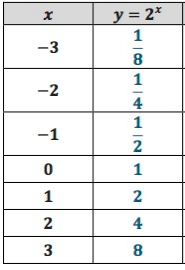# LT 5B Explore Exponential Functions

Author:
breeser

## A set of coordinates of an exponential function is given below.The table above represents the coordinates of the function  from x=-3 to x=3.
On the set of axes below, plot the points from the table above. Next, graph the diagonal line with equation 𝒚 = 𝒙. Finally. reflect each point across the diagonal line using the reflection tool.